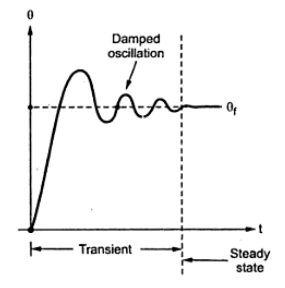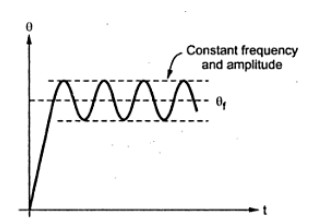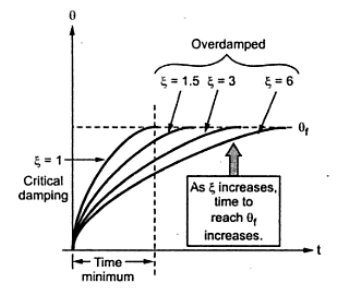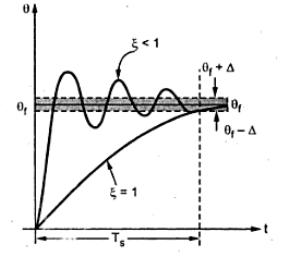### D'Arsonval Galvanometr

The use of D'Arsonval galvanometer is very common in variety of measuring instruments. The galvanometer is basically used in an instrument for detecting the presence of small voltages or currents in a circuit or to indicate zero current in applications like bridge circuits. Thus galvanometer has to be very much sensitive.
1.1 Construction
The construction of  D'Arsonval galvanometer is shown in the Fig. 1.Fig 1. Construction of D'Arsonval Galvanometr
It consists of the following parts,
1. Moving coil: The moving coil is rectangular or circular in cross-section, carrying number of turns of fine wire. It carries the current proportional to the quantity to be measured. It is suspended in the air gap between the poles of a permanent magnet and iron core. It is free to turn about its vertical axis. The pole faces are of particular shape such that the magnetic field is radial.
2. Iron core: It is spherical if coil is circular and cylindrical if coil is rectangular. It is basically used to provide low reluctance path the the magnetic flux and to produce strong magnetic field. This ensures higher deflecting torque and better sensitivity of  the galvanometer. The air gap is about 1/16 inches i.e. about 1.5 mm. If small moment of inertia is necessary, the iron core can be be omitted but it decreases the sensitivity.
3. Suspension: The suspension is a single file strip of phosphor-bronze and serves as one lead of the coil. The other lead takes the form of a loosely coiled spiral of fine wire leading downwards from the bottom of the coil. This is lower suspension. This type of galvanometer requires a perfect leveling so that the suspension coils remain straight and in central position without rubbing the poles or iron core. in galvanometers which do not require the perfect leveling, taut suspensions with straight flat strips are used, which are kept under tension from both sides.
4. Damping: The damping is eddy current damping. The eddy currents developed in the metal former on which coil is mounted, are responsible to produce damping torque. For effective damping a low resistance is connected across the galvanometer terminals. By adjusting the value of this resistance damping can be changed and critical damping can be achieved.
5. Indication: The suspension carries a small mirror upon which a beam of light is cast through a glass window in the outer brass case surrounding the instrument. The beam of light is reflected on the scale. The scale is usually 1m away from the mirror.
6. Zero adjustment: A torsion head is provided for the adjustment of the coil position and zero setting.
1.2 Torque Equation
The various parameters involved in torque are,
l  = Length of coil measured along vertical axis in m.
r = width of coil in m,
N = Number of turns of coil.
B = Flux density in air gap in Wb/m2 or Tesla.
i = Current through coil in A.
k = Spring constant or restoring constant in Nm/rad.
α  = Angle between plane of coil and direction of magnetic field.
A = Area of coil in m l x r.
F = Force on each side of a coil = NB ilsin N                 .....(1)
Td  = Deflecting torque = F x d = NB ilsinr                       ......(2)
...              Td = NB iA sin α
As the field is radial in nature, = 90o hence sinα = 1.
...               Td  = NB i A = Gi = G i Nm                               .......... (3)
where G = NBA = Galvanometer constant
The restoring torque provided by the spring is directly proportional to the final deflection of the coil.
Tc   = k θf
For final steady state position of coil,
Td   = Tc
The scale is calibrated in mm. The scale is at a distance of 1 m from the mirror as shown in the Fig.2.Fig.2 Measurement of deflection in mm

For small deflection, the radius of arc and angle of turning, decide the deflection.The angle through which the beam gets reflected is 2θif mirror is turned through θf.
Note: Usually r = 1 m = 1000 mm for the galvanometer.

1.3 Instrinsic Constants of Galvanometer
The various instrinsic constants of galvanometer are,
1. Displacement constant (G): The constant G defined in torque equation of a galvanometer is called displacement constant.
2. Constant of inertia (J): The inertia of system opposes the motion. Thus inertia produces a retarding torque given by

3. Damping constant: Another torque retarding the motion is friction in air and elastic hysteresis in the suspension. It is assumed to be proportional to the angular velocity of the moving system.
4. Control constant: The elasticity of the suspension is proportional to the displacement which produces controlling torque. This is required to bring the moving system back to the original position.
This constant is also called stiffness constant.
1.4 Dynamic Behaviour of Galvanometer
The dynamic behaviour of galvanometer is analysed through its equation of motion.Fig.3 Torques acts in galvanometer motion

This is second order differential equation governing the galvanometer motion. The solution of this equation has two parts, 1) Complementary function, 2) Particular integral.
The complementary function (C.F.) represents the transient behaviour while particular integral (P.I.) represents the steady state condition i.e. final deflection of the moving system.
The behaviour of system before it achieves the steady state is transient behaviour. When transient behaviour dies out, the system achieves final steady state position.
The auxiliary equation of above differential equation is obtained as,
Hence the solution has two exponential terms of power m1  and m2

where A, B = constants

Thus the complete solution is the addition of C.F. and P.I.
Now the transient terms may be purely exponential or oscillatory which depends on the nature of roots m1  and m1. This defines the various damping conditions of the system.
1.4.1 Underdamped Motion
Both m1  and m1 are complex conjugates of each other having negative real part.
Note: The transient behaviour is damped oscillations i.e. oscillations of decreasing amplitude. After sometimes amplitude becomes zero and system achieves steady state.
The roots are imaginary and complex conjugates of each other represented as,

Comparing equations (18) and (20),
Thus the final equation for underdamped case is,
The nature of such oscillations is shown in the Fig. 4.Fig.4 Underdamped motion galvanometer
For constant F and are to be obtained from initial conditions.
Obtaining F and α:
To obtain F and α i.e. P and Q use initial condition i.e. at t = 0 and θ = 0 in the equation (21),

Using equations (22) in equation (23),

Using equations (24) and (26) in equation (21),
The angular frequency is  ωhence
1.4.2 Undamped Motion
The motion existing when damping is made zero i.e. D = 0 is called undamped motion of the system. The roots are purely imaginary with real part for this case.
Note : The oscillations with zero damping are natural oscillations without position, having highest frequency. This frequency of undamped oscillations is called natural frequency of oscillations denoted as ωn.
Thus the solution of undamped motion is,

These oscillations are shown in the Fig.5.Fig. 5  Undamped motion of galvanometer
These are the oscillations with constant frequency and amplitude about the final position θf . Such oscillator are called sustained oscillations.
1.4.3 Critically Damped Motion
For critically damping, the roots  m1  and m2 are equal, real and negative. Thus D2 - 4 JK= 0 i.e D2 = 4JK  and D = 2 (JK) for critical damping.
Note: For this case, the transient response is not oscillatory but purely exponential such that pointer attains the steady state position very quickly.
As the response is not oscillatory, equation (28) is not applicable for this case.
The solution for the critically damped case is,

The value of damping constant for the critical damping is denoted  by Dc and Dc = (JK)
Using in equation (36),
1.4.4 Overdamped Motion
The amount of damping is mathematically measured by define a ratio of actual damping D and critical damping Dc. This is called a damping ratio and denoted by greek letter ξ this is also called relative damping.
Thus when the actual damping is more than the damping for critical case, the motion is called overdamped and the roots  m1  and mare real, unequal and negative.
Note: For overdamped case, the transient motion is purely exponential and nonoscillatory. The pointer attains final position θf  exponentially, taking more time than that of critical damping.
More the value damping, the pointer response is slow and sluggish, taking more time to attain the final position.
Note : For critical damping ξ = 1 while for overdamped case damping ratio ξ ˂ 1.
The solution for overdamped motion of is given as,
The pointer motion for critically damped and the overdamped case is shown in the Fig. 6.Fig. 6 Critically and overdamped motion
Note: As this motion is slow, practically overdamping is avoided in the instruments.
The critical damping is preferred practically for the instruments.

Table 1 Types of galvanometer motion
1.5 Logarithmic Decrement
Consider the underdamped galvanometer motion as shown in the Fig.7.Fig. 7 Overshoot and undershoot motion
The amount by which the pointer exceeds its final position, during first attempt is called the first overshoot. This is maximum in all the overshoots existing in the transient period.
Thus at t = t1, the deflection is maximum equal to θ1. According to maxima theorem, the time tat which deflection is maximum, must stasisfy dθ/dt|t=t1  = 0.
Rearrange equation (28) interms of ξ as,

This equation is satisfied when ωd t =  n because tan (nπ+θ) = tanθ.
...   t = (nπ)/ωd
for for first overshoot n  = 1, t = t1

The natural logarithm of the ratio of two successive swings is called logarithm decrement and denoted by λ.
where T= Time period corresponding to .
Thus equation (28) can be expressed as,
1.6 Setting Time
Te time required by the pointer to achieve steady state when the complete transient behaviour dies out is called setting time denoted as Ts.
Practically as the exponential term is present in the equation of motion, ξ is kept between 0.6 to 1 and motion is underdamped hence oscillatory. While a particular band is defined about θf denoted by ±Δ when transient oscillations decrease and enter into θf  ± Δ zone and remain thereafter within this interval then it is said the the pointer has achieved the steady state. This is shown in the Fig. 8.Fig. 8 Setting Time
For ± 2% band defined from accuracy point of view,
This is possible for ξ between 0.6 to 1.0 hence practically is designed between this range.
1.7 Effect of External Resistance on Damping
The damping means opposition to the motion by dissipating the energy of rotation. In galvanometer the damping is provided by two types,
1) Mechanical Damping: This is due to the friction present in the mechanical motion of the pointer. This is not very significant. The damping torque produced due to such mechanical effects is given by,
where Dm = Mechanical damping constant
2) Electromagnetic damping: This is effective damping than the mechanical damping. It is produced due to induced effects when coil moves in a magnetic field. Thus when coil moves in a magnetic field,
i) The eddy currents are induced in the metal former.
ii) The e.m.f. is induced in coil which circulates current through coil.
These effects cause damping called electromagnetic damping.
Let R = Resistance of galvanometer circuit = Rg+Rx
where Rg = Resistance of galvanometer coil
Rx = External resistance connected for damping
when coil rotates, e.m.f. is induced in it which is given by,

The torque produced due to this current flowing through te coil is,

Now let us find damping due to the metal former. It consists of one strip i.e. N=1.
where Rf   = Resistance of former
where Dformer   = Damping constant of former
where  D = DDr
1.7.1 Critical Resistance for Damping
The mechanical damping is very small and can be neglected
Practically the damping due to metal former is also very small

This is the value of external resistance required to adjust damping to the critical damping. It is called external critical damping resistance (ECDR).